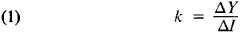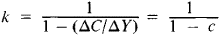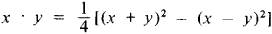# Multiplier

Also found in: Dictionary, Thesaurus, Legal, Financial, Acronyms, Wikipedia.
Related to Multiplier: multiplicand

## multiplier

1. a person or thing that multiplies
2. the number by which another number, the multiplicand is multiplied
3. Physics any device or instrument, such as a photomultiplier, for increasing an effect
4. Economics
a. the ratio of the total change in income (resulting from successive rounds of spending) to an initial autonomous change in expenditure
b. (as modifier): multiplier effects
Collins Discovery Encyclopedia, 1st edition © HarperCollins Publishers 2005
The following article is from The Great Soviet Encyclopedia (1979). It might be outdated or ideologically biased.

## Multiplier

in contemporary capitalist macroeconomics, a ratio that shows the dependence of change in national income on change in investment. With k representing the multiplier, ΔY = k Δ I where Δ Y and A ΔI express growth in national income and investment, respectively. The multiplier serves as a quantitative expression of the “principle of multiplication,” according to which every increase in employment directly related to autonomous investments—for example, state expenditures on public works—leads to growth in consumer demand, thus stimulating production in consumer goods sectors. This results in a further increase in consumer demand on the part of persons employed in other related sectors of production. This principle, proposed by R. F. Kahn in 1931 and developed in detail by J. M. Keynes, A. Hansen, P. Samuelson, and F. Machlup, was combined with the “principle of acceleration” to become part of the model of economic growth.

The multiplier depends directly on the “marginal propensity to consume” (C). This is defined as the ratio between growth in national income and expenditures on consumption resulting from this growth; it is measured by the fraction ΔC/Δ Y, where ΔC is growth in consumption and ΔY is growth in income.

If ΔY = k Δ I, thenBut proceeding from the equation ΔY = ΔC + Δ I and making the corresponding conversions in (1), we now have k = Δ Y/ (Δ Y —Δ C), orThe expression 1/(1 —c) is the multiplier. It can be replaced by using an algebraic expression for the marginal propensity to save, or s, which is defined as the ratio between growth in national income and that part of income that is saved. This is measured by the fraction ΔS/ Δ Y = s where ΔS is growth in savings and ΔK is growth in income. Then k = 1/s. Thus the multiplier is the inverse of the marginal propensity to save.

The rational elements of the idea of the multiplier lie in its reflection of real relationships between employment, savings, consumption, and national income. Among the concept’s basic flaws are the absence of socioeconomic content and the failure to analyze the real causes of the capitalist cycle. These flaws persist even while the concept of the multiplier represents an attempt to create an acceptable tool for countercrisis regulation of the capitalist economy, and thus to find a means of preserving the capitalist order during a period of economic depression. National income analysis is thus reduced to an assertion of the complete functional dependence of national income on investment at any given time, while change in national income depends on the total volume of investment for the current and preceding periods. The possibility of satisfying increased demand through increased labor productivity and technological progress is ignored. The real relationships between investment and national income are presented as depending upon a contrived “propensity to consume” that results from the operation of some “basic psychological law.” Thus it is claimed that these relationships are independent of the class structure of national income and consumption typical of capitalist society. The key role in all reproduction is ascribed to consumption, exchange, and distribution, not to production; from this it follows that it makes no difference whether the original increase in employment and income occurs in the production sphere or in the infrastructure. Even military expenditures are viewed as beneficial to the capitalist economy. In Marxist literature the theory of the multiplier is subjected to thorough criticism.

### REFERENCES

Keynes, J. M. Obshchaia teoriia zaniatosti, protsenta i deneg. Moscow, 1948. (Translated from English.)
Haberler, G. Protsvetanie i depressiia. Moscow, 1960. (Translated from English.)
Shliapentokh, V. E. Ekonometrika i problemy ekonomicheskogo rosta. Moscow, 1966.
Nosova, S. S. “Kritika teorii mul’tiplikatora i akseleratora.” In Kritika sovremennykh burzhuaznykh teorii finansov, deneg i kredita. Moscow, 1966. Pages 88–117.
Burachas, A. I. Teorii sprosa (Makroanaliz). Moscow, 1970.
Hicks, J. R. A Contribution to the Theory of the Trade Cycle. Oxford, 1950.

S. S. NOSOVA

## Multiplier

a device for increasing the rate of rotation of a shaft in a machine; it is made in the form of an independent mechanism, usually with step-up gearing. It is used infrequently, mainly in testing and laboratory equipment, when the speed of rotation of a motor that drives the shaft of a machine is insufficient to perform the required function.

## Multiplier

(or multiplier-divider), a computer component or a separate unit in which the operations of multiplication (division) are performed on quantities represented in analog or digital form.

In analog computers the operation of the multiplier is based on the hardware realization of physical and mathematical relationships that permit the conversion of input signals into an output signal proportional to the product of the inputs. Various relationships are made use of, including physical laws and phenomena, such as Ohm’s law and the Hall effect; the non-linearity of characteristics of electronic devices, for example, the nonlinear sector of the volt-ampere characteristic of a diode; and mathematical identity transformations that permit the operation of multiplication of two quantities to be replaced by other mathematical operations on the quantities, for example,or by operations on functions of the quantities, for example,

x · y = a[logax +loga y]

Also used are various electronic signal conversion methods, which can be described mathematically in terms of the multiplication of two values—for example, various types of modulation.

In digital computers the operation of multiplication is usually performed in the arithmetic unit. In specialized digital computers the multiplier is sometimes a separate functional unit. The matrix method of multiplication is most frequently used in this case: through a matrix of logic elements all the bit products are formed simultaneously and then summed. Tabular look-up is also used; here there are permanent memories that store, for example, tables of logarithms and antilogarithms. In this case, the codes of the factors are the addresses of the cells in which the factors’ logarithms are recorded. After the logarithms are summed, the address of the necessary cell of the table of antilogarithms is obtained; the result is read from there.

In hybrid computer systems both digital and analog techniques are used. For example, one of the factors in the form of a digital code is fed to the input of a digital-analog converter, while a second factor in analog form is used to regulate the reference voltage in the matrix of resistances. The result of the multiplication is obtained in the form of an analog value at the output of the converter.

### REFERENCES

Kazakov, V. A. Vychislitel’nye ustroistva mashin nepreryvnogo deistviia. Moscow, 1965.
Kartsev, M. A. Arifmetika tsifrovykh mashin. Moscow, 1969.
Gavrilov, Iu. V., and A. N. Puchko. Arifmeticheskie ustroistva bystrodeistvuiushchikh ETsVM. Moscow, 1970.
Computer Structures: Reading and Examples. New York, 1971.

E. A. SOKOLINSKII

## multiplier

[′məl·tə‚plī·ər]
(electricity)
A resistor used in series with a voltmeter to increase the voltage range. Also known as multiplier resistor.
(electronics)
A device that has two or more inputs and an output that is a representation of the product of the quantities represented by the input signals;voltages are the quantities commonly multiplied.
(mathematics)
If a number x is to be multiplied by a number y, then y is called the multiplier.
McGraw-Hill Dictionary of Scientific & Technical Terms, 6E, Copyright © 2003 by The McGraw-Hill Companies, Inc.

## multiplier

The factor by which an architect’s direct personnel expense is multiplied to determine compensation for his professional services or designated portions thereof.
McGraw-Hill Dictionary of Architecture and Construction. Copyright © 2003 by McGraw-Hill Companies, Inc.
References in periodicals archive ?
Dynamic multiplier effects of remittances in developing countries.
1, the multiplier is based on the equation [i.sub.out] = [square root of ([beta][I.sub.0])] [v.sub.i] where [I.sub.o] and [v.sub.i] are respectively the biasing current and input of the pair .
In authors have reported a fast and area efficient carry select adder, that is implemented for exponent addition in floating-point multiplier along with the parallel processing of various units used in the architecture.
Therefore, the multiplier applied to the initial upward camber caused by prestressing force is equal to be 1 + [[mu].sub.pe] = 1 + 0.85(0.925) = 1.80.
In that case, "the United States Supreme Court unequivocally repudiated the use of the contingency fee multiplier. The majority's decision here underscores the need for a full re-examination in a future case of our multiplier jurisprudence in light of the reasoning of Dague," Canady wrote.
As a result, we contribute further to the multiplier effect.
As shown in Fig.3, the proposed CW voltage multiplier is constructed by a flipped cascade connection of the stages.
The aim of this paper is to go beyond that characterization and prove a bijection between arbitrary multiplier bimonoids in C and arbitrary simplicial maps from C to a suitable simplicial set [M.sub.12].
In this section, we present a binary-decimal compression (BDC) based BCD multiplier. The proposed approach consists of 1 x 1-digit binary multiplication, partial product generation, binary-decimal compression, and decimal addition.
Hybrid Symmetrical Voltage Multiplier. Recently a new symmetrical voltage multiplier has been proposed .
In the case of zero lower bound (ZLB) interest rate situation the multiplier for output is >unity.

Site: Follow: Share:
Open / Close